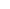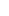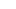# Forex Smart Trade Results August 15, 2022 – No Trade. Out With Covid###### Forex Smart Trade Results August 12, 2022 – \$1,340
August 26, 2022August 26, 2022

# Here’s how to calculate Leverage.

`Leverage = 1 / Margin Requirement`

For example, if the Margin Requirement is 2%, here’s how to calculate leverage:

`50 = 1 / .02`

The leverage is 50, which is expressed as a ratio, 50:1

Here’s how to calculate the Margin Requirement based on the Leverage Ratio:

`Margin Requirement = 1 / Leverage Ratio`

For example, if the Leverage Ratio is 100:1, here’s how to calculate the Margin Requirement.

`0.01 = 1 / 100`

The Margin Requirement is 0.01 or 1%.

As you can see, leverage has an inverse relationship to margin.

“Leverage” and “margin” refer to the same concept, just from a slightly different angle.

When a trader opens a position, they are required to put up a fraction of that position’s value “in good faith”. In this case, the trader is said to be “leveraged”.

The “fraction” part which is expressed in percentage terms is known as the “Margin Requirement”. For example, 2%.

The actual amount that is required to be put up is known as the “Required Margin”.

For example, 2% of a \$100,000 position size would be \$2,000.

The \$2,000 is the Required Margin to open this specific position.

Since you are able to trade a \$100,000 position size with just \$2,000, your leverage ratio is 50:1.

`Leverage = 1 /Margin Requirement 50 = 1 / 0.02`## Learn to Day Trade Forex

If you’d like to earn extra income trading on the Forex market, consider learning how to currency trade with Forex Smart Trade.   With their super-accurate proprietary trading tools and best-in-the-business, personalized one-on-one training, you’ll be successful.  Check out the Forex Smart Trade webinar.  It shows one of their trader’s trading and how easy, intuitive, and accurate the tools are.  Or try the Forex Smart Trade 14-day introductory trial for just TEN dollars.##### developer1